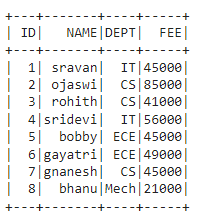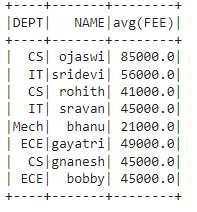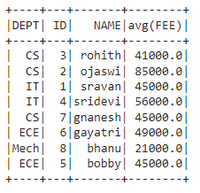GeeksforGeeks App
Open AppBrowser
Continue

# Pyspark – Aggregation on multiple columns

In this article, we will discuss how to perform aggregation on multiple columns in Pyspark using Python. We can do this by using Groupby() function

## Python3

 `# importing module``import` `pyspark`` ` `# importing sparksession from pyspark.sql module``from` `pyspark.sql ``import` `SparkSession`` ` `# creating sparksession and giving an app name``spark ``=` `SparkSession.builder.appName(``'sparkdf'``).getOrCreate()`` ` `# list  of student  data``data ``=` `[[``"1"``, ``"sravan"``, ``"IT"``, ``45000``],``        ``[``"2"``, ``"ojaswi"``, ``"CS"``, ``85000``],``        ``[``"3"``, ``"rohith"``, ``"CS"``, ``41000``],``        ``[``"4"``, ``"sridevi"``, ``"IT"``, ``56000``],``        ``[``"5"``, ``"bobby"``, ``"ECE"``, ``45000``],``        ``[``"6"``, ``"gayatri"``, ``"ECE"``, ``49000``],``        ``[``"7"``, ``"gnanesh"``, ``"CS"``, ``45000``],``        ``[``"8"``, ``"bhanu"``, ``"Mech"``, ``21000``]``        ``]`` ` `# specify column names``columns ``=` `[``'ID'``, ``'NAME'``, ``'DEPT'``, ``'FEE'``]`` ` `# creating a dataframe from the lists of data``dataframe ``=` `spark.createDataFrame(data, columns)`` ` `# display``dataframe.show()`

Output:In PySpark, groupBy() is used to collect the identical data into groups on the PySpark DataFrame and perform aggregate functions on the grouped data

### The aggregation operation includes:

• count(): This will return the count of rows for each group.

dataframe.groupBy(‘column_name_group’).count()

• mean(): This will return the mean of values for each group.

dataframe.groupBy(‘column_name_group’).mean(‘column_name’)

• max(): This will return the maximum of values for each group.

dataframe.groupBy(‘column_name_group’).max(‘column_name’)

• min(): This will return the minimum of values for each group.

dataframe.groupBy(‘column_name_group’).min(‘column_name’)

• sum(): This will return the total values for each group.

dataframe.groupBy(‘column_name_group’).sum(‘column_name’)

• avg(): This will return the average for values for each group.

dataframe.groupBy(‘column_name_group’).avg(‘column_name’).show()

We can   groupBy and aggregate on multiple columns at a time by using the following syntax:

dataframe.groupBy(‘column_name_group1′,’column_name_group2′,…………,’column_name_group n’).aggregate_operation(‘column_name’)

## Python3

 `# importing module``import` `pyspark`` ` `# importing sparksession from pyspark.sql module``from` `pyspark.sql ``import` `SparkSession`` ` `# creating sparksession and giving an app name``spark ``=` `SparkSession.builder.appName(``'sparkdf'``).getOrCreate()`` ` `# list  of student  data``data ``=` `[[``"1"``, ``"sravan"``, ``"IT"``, ``45000``],``        ``[``"2"``, ``"ojaswi"``, ``"CS"``, ``85000``],``        ``[``"3"``, ``"rohith"``, ``"CS"``, ``41000``],``        ``[``"4"``, ``"sridevi"``, ``"IT"``, ``56000``],``        ``[``"5"``, ``"bobby"``, ``"ECE"``, ``45000``],``        ``[``"6"``, ``"gayatri"``, ``"ECE"``, ``49000``],``        ``[``"7"``, ``"gnanesh"``, ``"CS"``, ``45000``],``        ``[``"8"``, ``"bhanu"``, ``"Mech"``, ``21000``]``        ``]`` ` `# specify column names``columns ``=` `[``'ID'``, ``'NAME'``, ``'DEPT'``, ``'FEE'``]`` ` `# creating a dataframe from the lists of data``dataframe ``=` `spark.createDataFrame(data, columns)`` ` `# Groupby with DEPT and NAME with mean()``dataframe.groupBy(``'DEPT'``, ``'NAME'``).mean(``'FEE'``).show()`

Output:Example 2: Aggregation on all columns

## Python3

 `# importing module``import` `pyspark`` ` `# importing sparksession from pyspark.sql module``from` `pyspark.sql ``import` `SparkSession`` ` `# creating sparksession and giving an app name``spark ``=` `SparkSession.builder.appName(``'sparkdf'``).getOrCreate()`` ` `# list  of student  data``data ``=` `[[``"1"``, ``"sravan"``, ``"IT"``, ``45000``],``        ``[``"2"``, ``"ojaswi"``, ``"CS"``, ``85000``],``        ``[``"3"``, ``"rohith"``, ``"CS"``, ``41000``],``        ``[``"4"``, ``"sridevi"``, ``"IT"``, ``56000``],``        ``[``"5"``, ``"bobby"``, ``"ECE"``, ``45000``],``        ``[``"6"``, ``"gayatri"``, ``"ECE"``, ``49000``],``        ``[``"7"``, ``"gnanesh"``, ``"CS"``, ``45000``],``        ``[``"8"``, ``"bhanu"``, ``"Mech"``, ``21000``]``        ``]`` ` `# specify column names``columns ``=` `[``'ID'``, ``'NAME'``, ``'DEPT'``, ``'FEE'``]`` ` `# creating a dataframe from the lists of data``dataframe ``=` `spark.createDataFrame(data, columns)`` ` `# Groupby with DEPT,ID and NAME with mean()``dataframe.groupBy(``'DEPT'``, ``'ID'``, ``'NAME'``).mean(``'FEE'``).show()`

Output:My Personal Notes arrow_drop_up